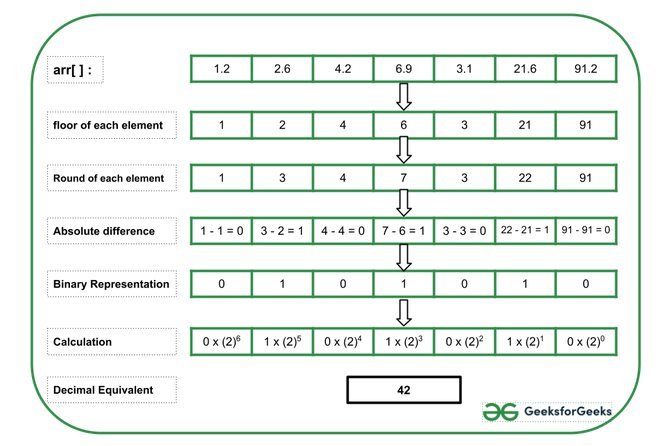Open in App
Not now

# Decimal equivalent of concatenation of absolute difference of floor and rounded-off values of array elements as a Binary String

• Last Updated : 12 Jul, 2021

Given an array arr[] consisting of N floating-point numbers, the task is to print the decimal representation of the binary array constructed from the absolute difference between the floor and round-off value for each array element.

Examples:

Input: arr[] = {1.2, 2.6, 4.2, 6.9, 3.1, 21.6, 91.2}
Output: 42
Explanation:
Below is the image to illustrate the above example:Input: arr[] = {5.7, 2.8, 1.9, 5.6, 2.2}
Output: 30

Approach: Follow the steps below to solve the problem:

• Initialize a variable, say result as 0 that stores the resultant numbers formed.
• Initialize a variable, say power as 0 that keeps the power of 2 added in each step.
• Traverse the given array arr[] from the end and perform the following steps:
• Initialize a variable, say bit that stores the absolute difference between the round-off value and the floor value of each array element.
• If the value of the absolute difference is 1, then multiply the digit with the proper power of 2 and add it to the variable result.
• Increment the value of power by 1.
• After completing the above steps, print the value of the result as the required decimal equivalent of the binary representation.

Below is the implementation of the above approach:

## C++

 `// C++ program for the above approach`   `#include ` `using` `namespace` `std;`   `// Function to find the decimal equivalent` `// of the new binary array constructed` `// from absolute decimal of floor and` `// the round-off values` `int` `findDecimal(``float` `arr[], ``int` `N)` `{` `    ``int` `bit, power = 0, result = 0;`   `    ``// Traverse the givenarray from` `    ``// the end` `    ``for` `(``int` `i = N - 1; i >= 0; i--) {`   `        ``// Stores the absolute difference` `        ``// between floor and round-off` `        ``// each array element` `        ``bit = ``abs``(``floor``(arr[i])` `                  ``- round(arr[i]));`   `        ``// If bit / difference is 1, then` `        ``// calculate the bit by proper` `        ``// power of 2 and add it to result` `        ``if` `(bit)` `            ``result += ``pow``(2, power);`   `        ``// Increment the value of power` `        ``power++;` `    ``}`   `    ``// Print the result` `    ``cout << result;` `}`   `// Driver Code` `int` `main()` `{` `    ``float` `arr[] = { 1.2, 2.6, 4.2, 6.9,` `                    ``3.1, 21.6, 91.2 };` `    ``int` `N = ``sizeof``(arr) / ``sizeof``(arr);` `    ``findDecimal(arr, N);`   `    ``return` `0;` `}`

## Java

 `// Java program for above approach` `import` `java.io.*;` `import` `java.lang.*;` `import` `java.util.*;`   `class` `GFG ` `{`   `// Function to find the decimal equivalent` `// of the new binary array constructed` `// from absolute decimal of floor and` `// the round-off values` `static` `void` `findDecimal(``double` `arr[], ``int` `N)` `{` `    ``int` `bit, power = ``0``, result = ``0``;` `  `  `    ``// Traverse the givenarray from` `    ``// the end` `    ``for` `(``int` `i = N - ``1``; i >= ``0``; i--)` `    ``{` `  `  `        ``// Stores the absolute difference` `        ``// between floor and round-off` `        ``// each array element` `        ``bit = Math.abs((``int``)Math.floor(arr[i])` `                  ``- (``int``)Math.round(arr[i]));` `  `  `        ``// If bit / difference is 1, then` `        ``// calculate the bit by proper` `        ``// power of 2 and add it to result` `        ``if` `(bit != ``0``)` `            ``result += Math.pow(``2``, power);` `  `  `        ``// Increment the value of power` `        ``power++;` `    ``}` `  `  `    ``// Print the result` `    ``System.out.print(result);` `}`   `    ``// Driver Code` `    ``public` `static` `void` `main(String[] args)` `    ``{` `    ``double` `arr[] = { ``1.2``, ``2.6``, ``4.2``, ``6.9``,` `                    ``3.1``, ``21.6``, ``91.2` `};` `    ``int` `N = arr.length;` `    ``findDecimal(arr, N);` `    ``}` `}`   `// This code is contributed by souravghosh0416.`

## Python3

 `# Python program for the above approach`     `# Function to find the decimal equivalent` `# of the new binary array constructed` `# from absolute decimal of floor and` `# the round-off values` `def` `findDecimal(arr, N):` `    ``power ``=` `0``;` `    ``result ``=` `0``;`   `    ``# Traverse the givenarray from` `    ``# the end` `    ``for` `i ``in` `range``(N ``-` `1``, ``-``1``, ``-``1``):`   `        ``# Stores the absolute difference` `        ``# between floor and round-off` `        ``# each array element` `        ``bit ``=` `abs``(``int``(arr[i]) ``-` `round``(arr[i]));`   `        ``# If bit / difference is 1, then` `        ``# calculate the bit by proper` `        ``# power of 2 and add it to result` `        ``if` `(bit):` `            ``result ``+``=` `pow``(``2``, power);`   `        ``# Increment the value of power` `        ``power ``+``=` `1``;`   `    ``# Print the result` `    ``print``(result);`     `# Driver Code`   `arr ``=` `[ ``1.2``, ``2.6``, ``4.2``, ``6.9``, ``3.1``, ``21.6``, ``91.2` `];` `N ``=` `len``(arr)` `findDecimal(arr, N);`   `# This code is contributed by gfgking.`

## C#

 `// C# program for the above approach` `using` `System;`   `class` `GFG{`   `// Function to find the decimal equivalent` `// of the new binary array constructed` `// from absolute decimal of floor and` `// the round-off values` `static` `void` `findDecimal(``double``[] arr, ``int` `N)` `{` `    ``int` `bit, power = 0, result = 0;` `   `  `    ``// Traverse the givenarray from` `    ``// the end` `    ``for``(``int` `i = N - 1; i >= 0; i--)` `    ``{` `   `  `        ``// Stores the absolute difference` `        ``// between floor and round-off` `        ``// each array element` `        ``bit = Math.Abs((``int``)Math.Floor(arr[i]) - ` `                       ``(``int``)Math.Round(arr[i]));` `   `  `        ``// If bit / difference is 1, then` `        ``// calculate the bit by proper` `        ``// power of 2 and add it to result` `        ``if` `(bit != 0)` `            ``result += (``int``)Math.Pow(2, power);` `   `  `        ``// Increment the value of power` `        ``power++;` `    ``}` `   `  `    ``// Print the result` `    ``Console.WriteLine(result);` `}` `    `  `// Driver Code` `public` `static` `void` `Main()` `{` `    ``double``[] arr = { 1.2, 2.6, 4.2, 6.9,` `                     ``3.1, 21.6, 91.2 };` `    ``int` `N = arr.Length;` `    `  `    ``findDecimal(arr, N);` `}` `}`   `// This code is contriobuted by sanjoy_62`

## Javascript

 ``

Output:

`42`

Time Complexity: O(N)
Auxiliary Space: O(1)

My Personal Notes arrow_drop_up
Related Articles